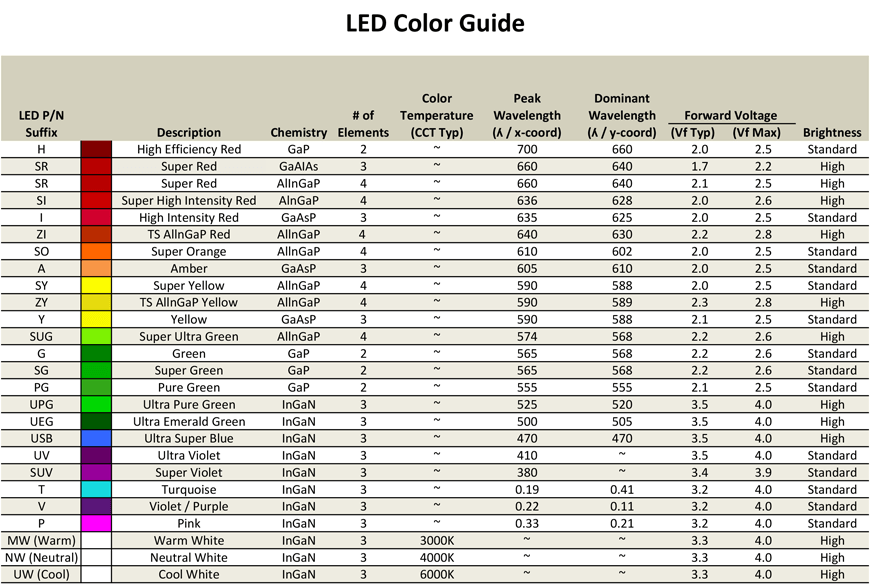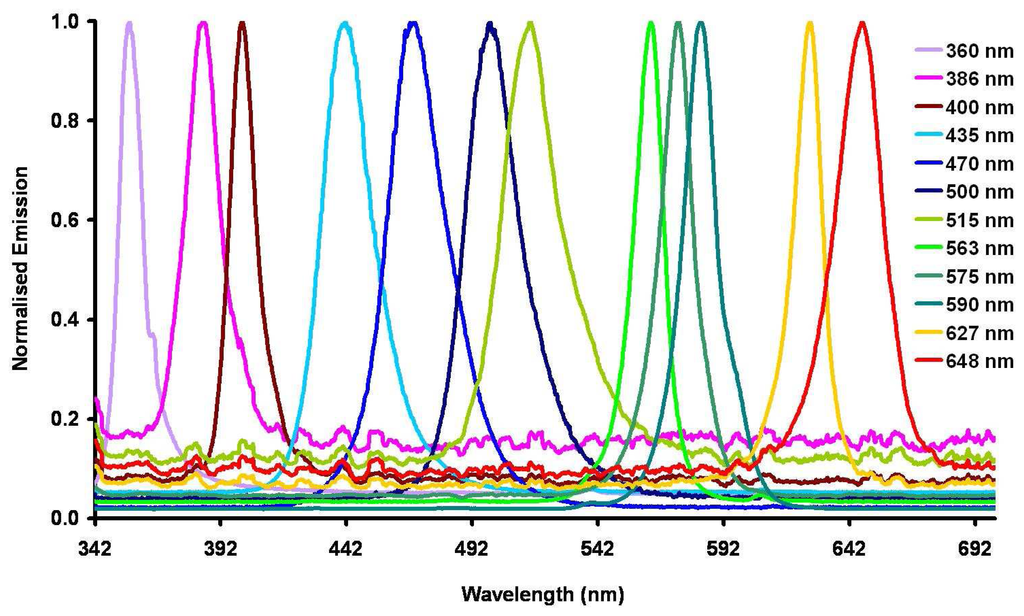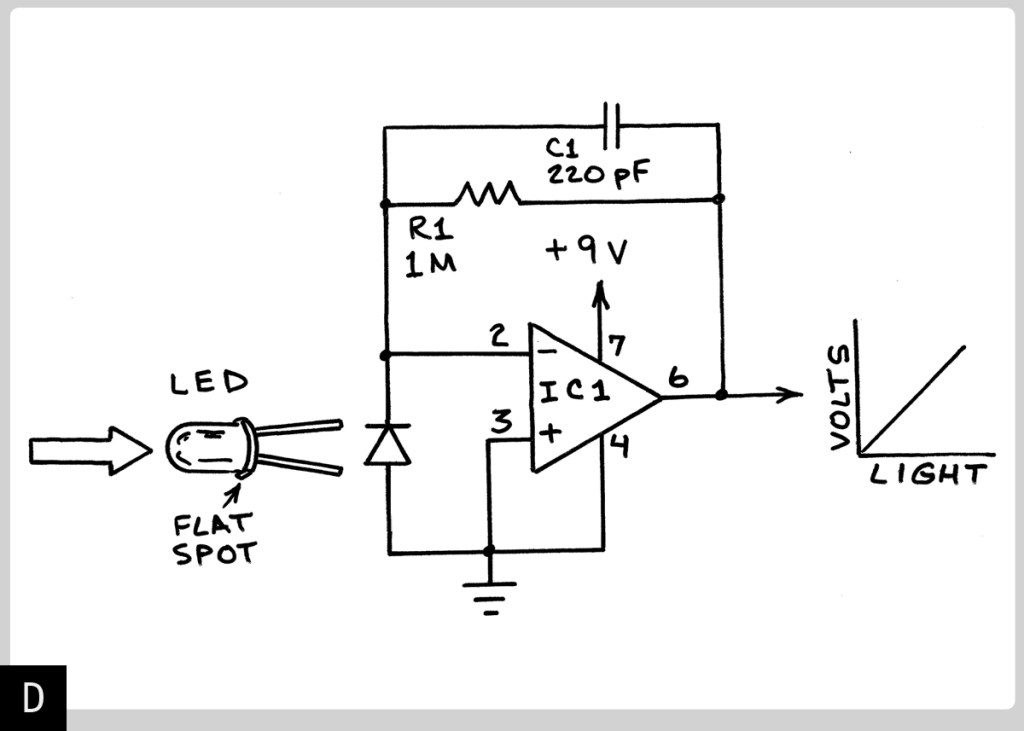# Mims effect

A microcontroller, e.g ESP32 or Arduino is capable to measure light received by a LED using one of their analog input pin or some GPIO directly without any circuit.

You can measure different types of LEDs or photoresistors, phototransistors, LDR, colour sensors, etc. The LED colour depends on chemical composition, different alloys containing aluminum, gallium, indium, phosphide, gallium nitride, etc.Emission table and graph of commercial LEDs

If you do no have a microcontroller at hand you can use inexpensive operational amplifiers, e.g.  Linear Technology LT1006 single-supply op-amp (IC1) provides a voltage output that’s almost perfectly linear with respect to the intensity of the incoming light. The gain or amplification equals the resistance of the feedback resistor (R1). Thus, when R1 is 1,000,000 ohms, the gain of the circuit is 1,000,000. Capacitor C1 prevents oscillation.CODE
//read analog data using Processing+Standard Firmata+Arduino
import processing.serial.*;
import cc.arduino.*;

Arduino arduino; //creates arduino object

color back = color(64, 218, 255); //variables for the 2 colors

int sensor= 0;

float value;

void setup() {
size(800, 600);
arduino = new Arduino(this, Arduino.list(), 57600); //sets up arduino
arduino.pinMode(sensor, Arduino.INPUT);//setup pins to be input (A0 =0?)

background(back);
}

void draw() {